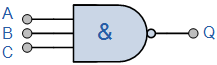The Logic NAND Gate is a combination of the digital logic AND gate with that of an inverter or NOT gate connected together in series.

The NAND (Not – AND) gate has an output that is normally at logic level “1” and only goes “LOW” to logic level “0” when ALL of its inputs are at logic level “1”. The Logic NAND Gate is the reverse or “Complementary” form of the AND gate we have seen previously.

Logic NAND Gate EquivalenceThe logic or Boolean expression given for a logic NAND gate is that for Logical Addition, which is the opposite to the AND gate, and which it performs on the complements of the inputs. The Boolean expression for a logic NAND gate is denoted by a single dot or full stop symbol, ( . ) with a line or Overline, ( ‾‾ ) over the expression to signify the NOT or logical negation of the NAND gate giving us the Boolean expression of:  A.B = Q.
Then we can define the operation of a 2-input digital logic NAND gate as being:
“If either A or B are NOT true, then Q is true”

A simple 2-input logic NAND gate can be constructed using RTL Resistor-transistor switches connected together as shown below with the inputs connected directly to the transistor bases. Either transistor must be cut-off “OFF” for an output at Q.Logic NAND Gates are available using digital circuits to produce the desired logical function and is given a symbol whose shape is that of a standard AND gate with a circle, sometimes called an “inversion bubble” at its output to represent the NOT gate symbol with the logical operation of the NAND gate given as.

The Digital Logic “NAND” Gate

Symbol               Truth Table3-input NAND Gate C B A Q 0 0 0 1 0 0 1 1 0 1 0 1 0 1 1 1 1 0 0 1 1 0 1 1 1 1 0 1 1 1 1 0 Boolean Expression Q = A.B.C Read as A AND B AND C gives NOT Q

As with the AND function seen previously, the NAND function can also have any number of individual inputs and commercial available NAND Gate IC’s are available in standard 2, 3, or 4 input types. If additional inputs are required, then the standard NAND gates can be cascaded together to provide more inputs for example.

A 4-input NAND FunctionThe Boolean Expression for this 4-input logic NAND gate will therefore be:   Q = A.B.C.D
If the number of inputs required is an odd number of inputs any “unused” inputs can be held HIGH by connecting them directly to the power supply using suitable “Pull-up” resistors.
The Logic NAND Gate function is sometimes known as the Sheffer Stroke Function and is denoted by a vertical bar or upwards arrow operator, for example, A NAND B = A|B or AB.

The “Universal” NAND Gate

The Logic NAND Gate is generally classed as a “Universal” gate because it is one of the most commonly used logic gate types. NAND gates can also be used to produce any other type of logic gate function, and in practice the NAND gate forms the basis of most practical logic circuits. By connecting them together in various combinations the three basic gate types of AND, OR and NOT function can be formed using only NAND‘s, for example.

Various Logic Gates using only NAND GatesAs well as the three common types above, Ex-Or, Ex-Nor and standard NOR gates can be formed using just individual NAND gates.
Commonly available digital logic NAND gate IC’s include:
TTL Logic NAND Gates
• 74LS10 Triple 3-input
• 74LS20 Dual 4-input
• 74LS30 Single 8-input
CMOS Logic NAND Gates